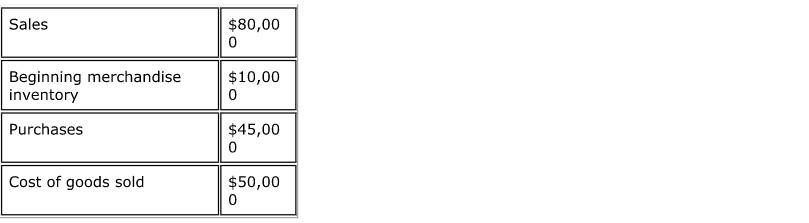# Problem: Consider the following: Determine the value of the ending merchandise inventory. a. $10,000 b.$15,000 c. $5,000 d.$25,000

###### Problem Details

Consider the following:

Determine the value of the ending merchandise inventory.

a. $10,000 b.$15,000

c. $5,000 d.$25,000